# Mean State

Period Mean (original grids) [K]
Model Period Mean (intersection) [K]
Model Period Mean (complement) [K]
Benchmark Period Mean (intersection) [K]
Benchmark Period Mean (complement) [K]
Bias [K]
RMSE [K]
Phase Shift [months]
Bias Score 
RMSE Score 
Seasonal Cycle Score 
Spatial Distribution Score 
Interannual Variability Score 
Overall Score 
Benchmark [-] 299.
CRUNCEPv7 [-] 299. 299. 301. 298. 300. 0.167 0.744 0.331 0.62 0.79 0.96 0.98 0.96 0.85
GSWP3v1 [-] 299. 299. 300. 298. 300. 0.273 0.683 0.342 0.67 0.78 0.97 1.0 0.96 0.86
WFDEI [-] 299. 299. 301. 298. 300. 0.327 1.03 0.426 0.61 0.52 0.96 0.99 0.88 0.75
Period Mean (original grids) [K]
Model Period Mean (intersection) [K]
Model Period Mean (complement) [K]
Benchmark Period Mean (intersection) [K]
Benchmark Period Mean (complement) [K]
Bias [K]
RMSE [K]
Phase Shift [months]
Bias Score 
RMSE Score 
Seasonal Cycle Score 
Spatial Distribution Score 
Interannual Variability Score 
Overall Score 
Benchmark [-] 300.
CRUNCEPv7 [-] 301. 301. 301. 300. 300. 0.445 0.818 0.178 0.75 0.85 0.99 1.0 0.97 0.90
GSWP3v1 [-] 301. 301. 301. 300. 300. 0.412 0.773 0.166 0.76 0.86 0.99 0.99 0.96 0.90
WFDEI [-] 300. 300. 301. 300. 300. 0.266 1.33 0.258 0.77 0.62 0.98 1.0 0.91 0.82
Period Mean (original grids) [K]
Model Period Mean (intersection) [K]
Model Period Mean (complement) [K]
Benchmark Period Mean (intersection) [K]
Benchmark Period Mean (complement) [K]
Bias [K]
RMSE [K]
Phase Shift [months]
Bias Score 
RMSE Score 
Seasonal Cycle Score 
Spatial Distribution Score 
Interannual Variability Score 
Overall Score 
Benchmark [-] 295.
CRUNCEPv7 [-] 296. 296. 297. 295. 297. 0.455 0.854 0.360 0.71 0.86 0.96 1.0 0.95 0.89
GSWP3v1 [-] 296. 296. 296. 295. 297. 0.471 0.825 0.298 0.72 0.87 0.96 1.0 0.96 0.90
WFDEI [-] 295. 295. 297. 295. 297. 0.295 1.14 0.480 0.73 0.69 0.94 1.0 0.88 0.82
Period Mean (original grids) [K]
Model Period Mean (intersection) [K]
Model Period Mean (complement) [K]
Benchmark Period Mean (intersection) [K]
Benchmark Period Mean (complement) [K]
Bias [K]
RMSE [K]
Phase Shift [months]
Bias Score 
RMSE Score 
Seasonal Cycle Score 
Spatial Distribution Score 
Interannual Variability Score 
Overall Score 
Benchmark [-] 262.
CRUNCEPv7 [-] 260. 260. 263. 262. 266. -1.03 1.64 0.0159 0.92 0.94 1.0 1.0 0.92 0.95
GSWP3v1 [-] 262. 261. 267. 262. 266. 0.243 1.14 0.0153 0.94 0.96 1.0 1.0 0.94 0.96
WFDEI [-] 260. 260. 263. 262. 266. -1.58 3.38 0.0269 0.90 0.83 1.0 1.0 0.92 0.91
Period Mean (original grids) [K]
Model Period Mean (intersection) [K]
Model Period Mean (complement) [K]
Benchmark Period Mean (intersection) [K]
Benchmark Period Mean (complement) [K]
Bias [K]
RMSE [K]
Phase Shift [months]
Bias Score 
RMSE Score 
Seasonal Cycle Score 
Spatial Distribution Score 
Interannual Variability Score 
Overall Score 
Benchmark [-] 299.
CRUNCEPv7 [-] 299. 299. 300. 299. 299. -0.00747 0.779 0.604 0.42 0.69 0.92 1.0 0.88 0.76
GSWP3v1 [-] 299. 299. 300. 299. 299. 0.194 0.749 0.487 0.43 0.72 0.93 0.96 0.91 0.78
WFDEI [-] 299. 299. 300. 299. 299. 0.338 0.948 0.886 0.39 0.41 0.86 0.99 0.87 0.65
Period Mean (original grids) [K]
Model Period Mean (intersection) [K]
Model Period Mean (complement) [K]
Benchmark Period Mean (intersection) [K]
Benchmark Period Mean (complement) [K]
Bias [K]
RMSE [K]
Phase Shift [months]
Bias Score 
RMSE Score 
Seasonal Cycle Score 
Spatial Distribution Score 
Interannual Variability Score 
Overall Score 
Benchmark [-] 271.
CRUNCEPv7 [-] 271. 270. 274. 271. 274. -0.709 1.06 0.00815 0.94 0.96 1.0 1.0 0.98 0.97
GSWP3v1 [-] 271. 271. 275. 271. 274. -0.00239 0.633 0.00842 0.97 0.97 1.0 1.0 0.98 0.98
WFDEI [-] 270. 270. 273. 271. 274. -1.29 3.32 0.00841 0.91 0.80 1.0 1.0 0.92 0.91
Period Mean (original grids) [K]
Model Period Mean (intersection) [K]
Model Period Mean (complement) [K]
Benchmark Period Mean (intersection) [K]
Benchmark Period Mean (complement) [K]
Bias [K]
RMSE [K]
Phase Shift [months]
Bias Score 
RMSE Score 
Seasonal Cycle Score 
Spatial Distribution Score 
Interannual Variability Score 
Overall Score 
Benchmark [-] 280.
CRUNCEPv7 [-] 280. 280. 287. 280. 285. 0.0112 1.17 0.0268 0.90 0.95 1.0 1.0 0.96 0.96
GSWP3v1 [-] 281. 280. 288. 280. 285. 0.317 1.10 0.0205 0.90 0.96 1.0 1.0 0.97 0.96
WFDEI [-] 280. 280. 287. 280. 285. -0.284 2.48 0.0400 0.89 0.82 1.0 1.0 0.91 0.91
Period Mean (original grids) [K]
Model Period Mean (intersection) [K]
Model Period Mean (complement) [K]
Benchmark Period Mean (intersection) [K]
Benchmark Period Mean (complement) [K]
Bias [K]
RMSE [K]
Phase Shift [months]
Bias Score 
RMSE Score 
Seasonal Cycle Score 
Spatial Distribution Score 
Interannual Variability Score 
Overall Score 
Benchmark [-] 297.
CRUNCEPv7 [-] 297. 297. 300. 297. 300. 0.186 1.14 0.178 0.75 0.88 0.98 1.0 0.94 0.90
GSWP3v1 [-] 297. 297. 300. 297. 300. 0.0966 1.09 0.168 0.77 0.87 0.98 1.0 0.95 0.91
WFDEI [-] 297. 297. 300. 297. 300. 0.140 1.54 0.201 0.76 0.73 0.98 1.0 0.92 0.85
Period Mean (original grids) [K]
Model Period Mean (intersection) [K]
Model Period Mean (complement) [K]
Benchmark Period Mean (intersection) [K]
Benchmark Period Mean (complement) [K]
Bias [K]
RMSE [K]
Phase Shift [months]
Bias Score 
RMSE Score 
Seasonal Cycle Score 
Spatial Distribution Score 
Interannual Variability Score 
Overall Score 
Benchmark [-] 264.
CRUNCEPv7 [-] 263. 263. 264. 264. 264. -1.17 1.53 0.0147 0.92 0.94 1.0 1.0 0.97 0.96
GSWP3v1 [-] 264. 264. 267. 264. 264. 0.0228 0.741 0.0121 0.97 0.96 1.0 1.0 0.96 0.98
WFDEI [-] 262. 262. 263. 264. 264. -1.68 4.03 0.0104 0.89 0.78 1.0 1.0 0.96 0.90
Period Mean (original grids) [K]
Model Period Mean (intersection) [K]
Model Period Mean (complement) [K]
Benchmark Period Mean (intersection) [K]
Benchmark Period Mean (complement) [K]
Bias [K]
RMSE [K]
Phase Shift [months]
Bias Score 
RMSE Score 
Seasonal Cycle Score 
Spatial Distribution Score 
Interannual Variability Score 
Overall Score 
Benchmark [-] 295.
CRUNCEPv7 [-] 295. 295. 293. 295. 294. 0.337 0.625 0.0795 0.89 0.93 0.99 1.0 0.95 0.95
GSWP3v1 [-] 295. 295. 294. 295. 294. 0.502 0.712 0.0784 0.87 0.93 0.99 1.0 0.97 0.95
WFDEI [-] 295. 295. 293. 295. 294. 0.415 1.49 0.150 0.87 0.76 0.99 1.0 0.93 0.88
Period Mean (original grids) [K]
Model Period Mean (intersection) [K]
Model Period Mean (complement) [K]
Benchmark Period Mean (intersection) [K]
Benchmark Period Mean (complement) [K]
Bias [K]
RMSE [K]
Phase Shift [months]
Bias Score 
RMSE Score 
Seasonal Cycle Score 
Spatial Distribution Score 
Interannual Variability Score 
Overall Score 
Benchmark [-] 282.
CRUNCEPv7 [-] 282. 282. 284. 282. 286. -0.0854 0.985 0.106 0.89 0.92 0.99 1.0 0.95 0.95
GSWP3v1 [-] 282. 282. 284. 282. 286. 0.167 0.867 0.113 0.90 0.94 0.99 1.0 0.97 0.96
WFDEI [-] 281. 281. 283. 282. 286. -0.875 2.55 0.211 0.84 0.73 0.99 0.99 0.96 0.87
Period Mean (original grids) [K]
Model Period Mean (intersection) [K]
Model Period Mean (complement) [K]
Benchmark Period Mean (intersection) [K]
Benchmark Period Mean (complement) [K]
Bias [K]
RMSE [K]
Phase Shift [months]
Bias Score 
RMSE Score 
Seasonal Cycle Score 
Spatial Distribution Score 
Interannual Variability Score 
Overall Score 
Benchmark [-] 287.
CRUNCEPv7 [-] 282. 286. 244. 287. 292. -0.0764 1.03 0.150 0.82 0.90 0.98 1.0 0.95 0.93
GSWP3v1 [-] 283. 287. 249. 287. 292. 0.241 0.901 0.136 0.83 0.91 0.99 1.0 0.96 0.93
WFDEI [-] 282. 286. 247. 287. 292. -0.298 2.09 0.209 0.81 0.72 0.98 1.0 0.91 0.86
Period Mean (original grids) [K]
Model Period Mean (intersection) [K]
Model Period Mean (complement) [K]
Benchmark Period Mean (intersection) [K]
Benchmark Period Mean (complement) [K]
Bias [K]
RMSE [K]
Phase Shift [months]
Bias Score 
RMSE Score 
Seasonal Cycle Score 
Spatial Distribution Score 
Interannual Variability Score 
Overall Score 
Benchmark [-] 296.
CRUNCEPv7 [-] 296. 296. 297. 296. 297. 0.266 0.870 0.130 0.89 0.94 0.99 1.0 0.96 0.95
GSWP3v1 [-] 296. 296. 296. 296. 297. 0.213 0.830 0.134 0.90 0.94 0.99 1.0 0.96 0.95
WFDEI [-] 296. 296. 297. 296. 297. -0.179 1.70 0.193 0.89 0.80 0.98 1.0 0.91 0.90
Period Mean (original grids) [K]
Model Period Mean (intersection) [K]
Model Period Mean (complement) [K]
Benchmark Period Mean (intersection) [K]
Benchmark Period Mean (complement) [K]
Bias [K]
RMSE [K]
Phase Shift [months]
Bias Score 
RMSE Score 
Seasonal Cycle Score 
Spatial Distribution Score 
Interannual Variability Score 
Overall Score 
Benchmark [-] 265.
CRUNCEPv7 [-] 264. 264. 261. 265. 266. -0.968 1.47 0.0112 0.92 0.93 1.0 1.0 0.94 0.95
GSWP3v1 [-] 265. 265. 266. 265. 266. 0.264 0.995 0.0182 0.94 0.95 1.0 1.0 0.96 0.97
WFDEI [-] 264. 264. 261. 265. 266. -1.37 3.46 0.0347 0.90 0.79 1.0 0.99 0.92 0.90
Period Mean (original grids) [K]
Model Period Mean (intersection) [K]
Model Period Mean (complement) [K]
Benchmark Period Mean (intersection) [K]
Benchmark Period Mean (complement) [K]
Bias [K]
RMSE [K]
Phase Shift [months]
Bias Score 
RMSE Score 
Seasonal Cycle Score 
Spatial Distribution Score 
Interannual Variability Score 
Overall Score 
Benchmark [-] 296.
CRUNCEPv7 [-] 295. 295. 298. 296. 298. 0.180 1.21 0.275 0.67 0.84 0.98 1.0 0.92 0.88
GSWP3v1 [-] 296. 295. 298. 296. 298. 0.383 1.19 0.243 0.67 0.86 0.98 1.0 0.94 0.88
WFDEI [-] 295. 295. 298. 296. 298. 0.174 1.62 0.259 0.68 0.67 0.98 1.0 0.91 0.82
Period Mean (original grids) [K]
Model Period Mean (intersection) [K]
Model Period Mean (complement) [K]
Benchmark Period Mean (intersection) [K]
Benchmark Period Mean (complement) [K]
Bias [K]
RMSE [K]
Phase Shift [months]
Bias Score 
RMSE Score 
Seasonal Cycle Score 
Spatial Distribution Score 
Interannual Variability Score 
Overall Score 
Benchmark [-] 286.
CRUNCEPv7 [-] 286. 286. 294. 286. 292. -0.0108 0.672 0.0324 0.94 0.95 1.0 1.0 0.97 0.97
GSWP3v1 [-] 286. 286. 294. 286. 292. 0.305 0.619 0.0320 0.94 0.96 1.0 1.0 0.97 0.97
WFDEI [-] 286. 286. 293. 286. 292. -0.485 2.40 0.0561 0.93 0.77 1.0 1.0 0.95 0.90
Period Mean (original grids) [K]
Model Period Mean (intersection) [K]
Model Period Mean (complement) [K]
Benchmark Period Mean (intersection) [K]
Benchmark Period Mean (complement) [K]
Bias [K]
RMSE [K]
Phase Shift [months]
Bias Score 
RMSE Score 
Seasonal Cycle Score 
Spatial Distribution Score 
Interannual Variability Score 
Overall Score 
Benchmark [-] 275.
CRUNCEPv7 [-] 274. 274. 277. 274. 278. -0.539 1.01 0.0411 0.93 0.94 1.0 1.0 0.97 0.96
GSWP3v1 [-] 274. 274. 278. 274. 278. 0.149 0.698 0.0342 0.94 0.96 1.0 1.0 0.97 0.97
WFDEI [-] 273. 273. 277. 274. 278. -0.935 3.16 0.0431 0.90 0.76 1.0 0.99 0.92 0.89
Period Mean (original grids) [K]
Model Period Mean (intersection) [K]
Model Period Mean (complement) [K]
Benchmark Period Mean (intersection) [K]
Benchmark Period Mean (complement) [K]
Bias [K]
RMSE [K]
Phase Shift [months]
Bias Score 
RMSE Score 
Seasonal Cycle Score 
Spatial Distribution Score 
Interannual Variability Score 
Overall Score 
Benchmark [-] 284.
CRUNCEPv7 [-] 283. 283. 287. 284. 286. -0.0210 1.17 0.0285 0.88 0.93 1.0 1.0 0.96 0.95
GSWP3v1 [-] 284. 284. 287. 284. 286. 0.409 1.12 0.0285 0.88 0.94 1.0 1.0 0.96 0.95
WFDEI [-] 283. 283. 287. 284. 286. -0.0950 2.58 0.0334 0.88 0.76 1.0 1.0 0.91 0.88
Period Mean (original grids) [K]
Model Period Mean (intersection) [K]
Model Period Mean (complement) [K]
Benchmark Period Mean (intersection) [K]
Benchmark Period Mean (complement) [K]
Bias [K]
RMSE [K]
Phase Shift [months]
Bias Score 
RMSE Score 
Seasonal Cycle Score 
Spatial Distribution Score 
Interannual Variability Score 
Overall Score 
Benchmark [-] 299.
CRUNCEPv7 [-] 299. 299. 300. 299. 299. -0.0135 0.720 0.433 0.65 0.77 0.94 1.0 0.94 0.84
GSWP3v1 [-] 299. 299. 300. 299. 299. 0.144 0.683 0.388 0.66 0.78 0.95 1.0 0.93 0.85
WFDEI [-] 299. 299. 300. 299. 299. 0.158 1.11 0.683 0.63 0.43 0.90 1.0 0.90 0.72
Period Mean (original grids) [K]
Model Period Mean (intersection) [K]
Model Period Mean (complement) [K]
Benchmark Period Mean (intersection) [K]
Benchmark Period Mean (complement) [K]
Bias [K]
RMSE [K]
Phase Shift [months]
Bias Score 
RMSE Score 
Seasonal Cycle Score 
Spatial Distribution Score 
Interannual Variability Score 
Overall Score 
Benchmark [-] 296.
CRUNCEPv7 [-] 296. 296. 296. 296. 293. 0.323 0.668 0.267 0.77 0.87 0.97 1.0 0.94 0.90
GSWP3v1 [-] 296. 296. 296. 296. 293. 0.417 0.667 0.229 0.77 0.88 0.97 1.0 0.96 0.91
WFDEI [-] 296. 296. 296. 296. 293. 0.111 1.26 0.304 0.80 0.63 0.97 1.0 0.93 0.83
Period Mean (original grids) [K]
Model Period Mean (intersection) [K]
Model Period Mean (complement) [K]
Benchmark Period Mean (intersection) [K]
Benchmark Period Mean (complement) [K]
Bias [K]
RMSE [K]
Phase Shift [months]
Bias Score 
RMSE Score 
Seasonal Cycle Score 
Spatial Distribution Score 
Interannual Variability Score 
Overall Score 
Benchmark [-] 284.
CRUNCEPv7 [-] 284. 284. 288. 284. 285. 0.170 1.53 0.209 0.67 0.86 0.97 1.0 0.94 0.88
GSWP3v1 [-] 285. 284. 288. 284. 285. 0.552 1.57 0.184 0.66 0.86 0.98 1.0 0.95 0.88
WFDEI [-] 285. 284. 288. 284. 285. 0.546 2.12 0.268 0.65 0.68 0.97 1.0 0.93 0.82

# Temporally integrated period mean

BENCHMARK MEAN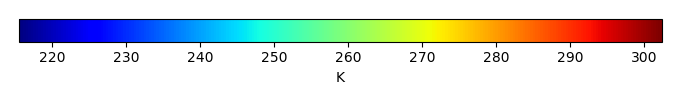MODEL MEANBIAS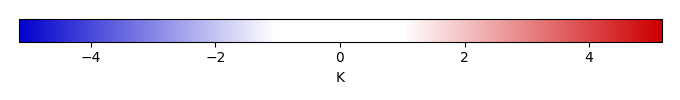BIAS SCORERMSE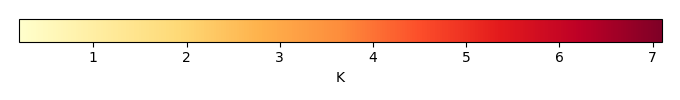RMSE SCOREBENCHMARK INTERANNUAL VARIABILITY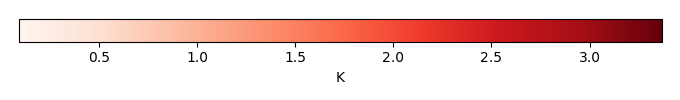MODEL INTERANNUAL VARIABILITYINTERANNUAL VARIABILITY SCOREBENCHMARK MAX MONTHMODEL MAX MONTHDIFFERENCE IN MAX MONTH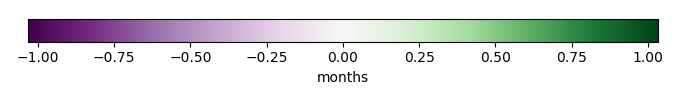SEASONAL CYCLE SCORESPATIAL TAYLOR DIAGRAMMODEL COLORS# Spatially integrated regional mean

MODEL COLORSREGIONAL MEANANNUAL CYCLEMONTHLY ANOMALYANNUAL CYCLE# All Models

BenchmarkCRUNCEPv7GSWP3v1WFDEI# Data Information

creation_date: Fri Nov 1 11:52:30 PDT 2013

source_file: This product is generated from monthly CRU TS3.21 Mean Temperature observations

title: derived CRU high resolution (0.5x0.5) Mean Temperature

Approach: I simply read the monthly surface air temperature from original file, and separated the time series by a month and saved them by each month and each year.

Temporal resolution: monthly

General information: This product was derived from CRU v3.21 product.

Spatial resolution: 0.5x0.5 degree